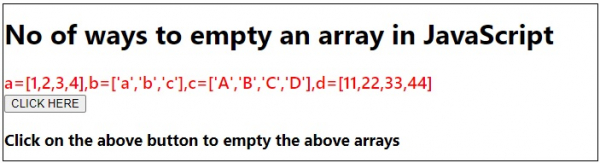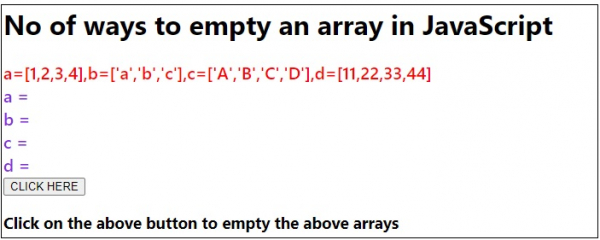# No. of ways to empty an array in JavaScript

To empty an array in JavaScript, there are four ways −

• Setting to new Array − In this we set our array variable to a new empty array.
• Using length property − In this we set the length property of our array to 0.
• Using pop − In this we continuously pop the array elements till the length reaches to 0.
• Using splice − In this we put the start index as 0 and the no of elements to removed as array.length-1.

Following is the code to display no. of ways to empty an array in JavaScript −

## Example

Live Demo

<!DOCTYPE html>
<html lang="en">
<meta charset="UTF-8" />
<meta name="viewport" content="width=device-width, initial-scale=1.0" />
<title>Document</title>
<style>
body {
font-family: "Segoe UI", Tahoma, Geneva, Verdana, sans-serif;
}
.result,
.sample {
font-size: 20px;
font-weight: 500;
color: blueviolet;
}
.sample {
color: red;
}
</style>
<body>
<h1>No of ways to empty an array in JavaScript</h1>
<div class="sample">
a=[1,2,3,4],b=['a','b','c'],c=['A','B','C','D'],d=[11,22,33,44]
</div>
<div class="result"></div>
<h3>Click on the above button to empty the above arrays</h3>
<script>
let resEle = document.querySelector(".result");
let a, b, c, d;
(a = [1, 2, 3, 4]),
(b = ["a", "b", "c"]),
(c = ["A", "B", "C", "D"]),
(d = [11, 22, 33, 44]);
let emptyArr = [];
a = emptyArr;
resEle.innerHTML = " a = " + a + "<br>";
b.length = 0;
resEle.innerHTML += " b = " + b + "<br>";
while (c.length > 0) {
c.pop();
}
resEle.innerHTML += "c = " + c + "<br>";
d.splice(0, d.length);
resEle.innerHTML += "d = " + d + "<br>";
});
</script>
</body>
</html>

## Output